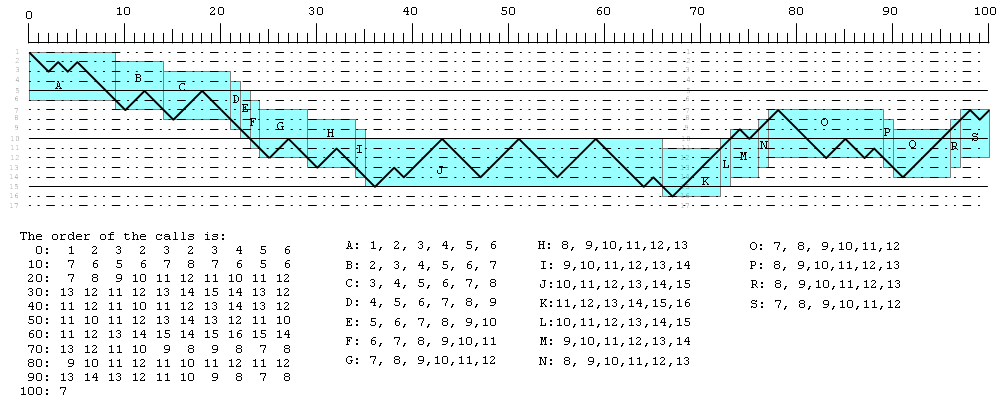This problem references Fig 4.21, on pg 154.

The idea behind this problem is the windowing system of a RISC computer, which is shown in Fig 15.13, pg 564. In that figure, the window size is 8, ie, there are 8 register windows. It is also rather confusing that the problem talks about a window size, when they really seem to mean the number of register windows. Normally, one would think a window size is the number of registers in a window, but that doesn't seem to be the interpretation being used in this problem. The problem is rather interesting when one makes this adjustment, which is how I am approaching this problem and Fig 4.21.

The first problem is that the figure is a mess and does not actually read correctly - at the very least there seem to be too many wiggles in the middle section. After some effort, I came up with the following figure, which is what I think they really had in mind:I have labelled the windows to make it easier to talk about the details in the figure.

The second problem is that the figure seems to be using a window size of 6 rather than 5, as I have shown in the table where I have indicated exactly which functions are currently in the set of active windows.

The answer given in the answer book for 15.1.a is 18, which is the number of boxes shown in Fig 4.21 - but the only reasonable interpretation of the figure is that the number of windows is 6, not 5. I'll go with that also.

I think you can now derive the answers for the other values of the number of register windows.

This is a very interesting problem, but it really needs to be explained much better, and without the listing of the calls as I have shown, the figure is just too ambiguous.

ND.